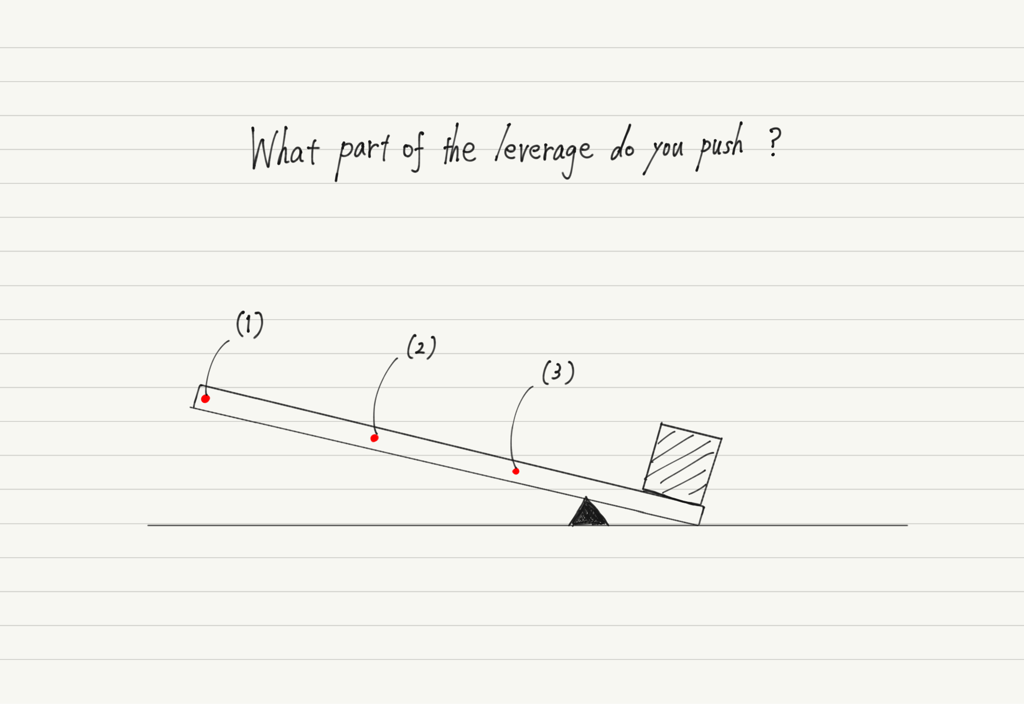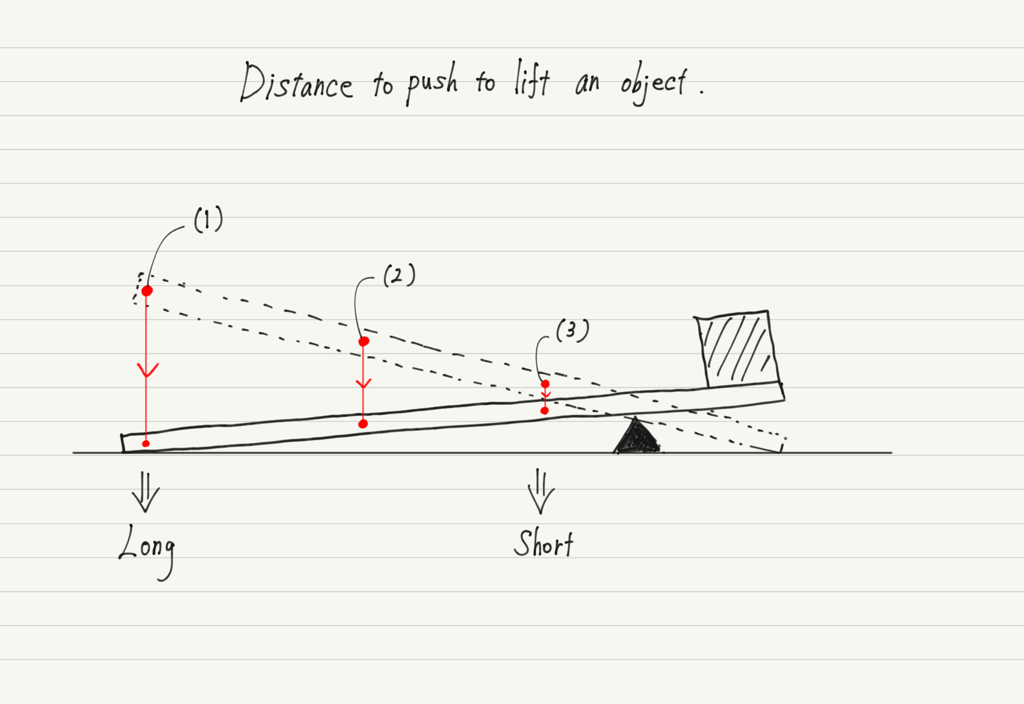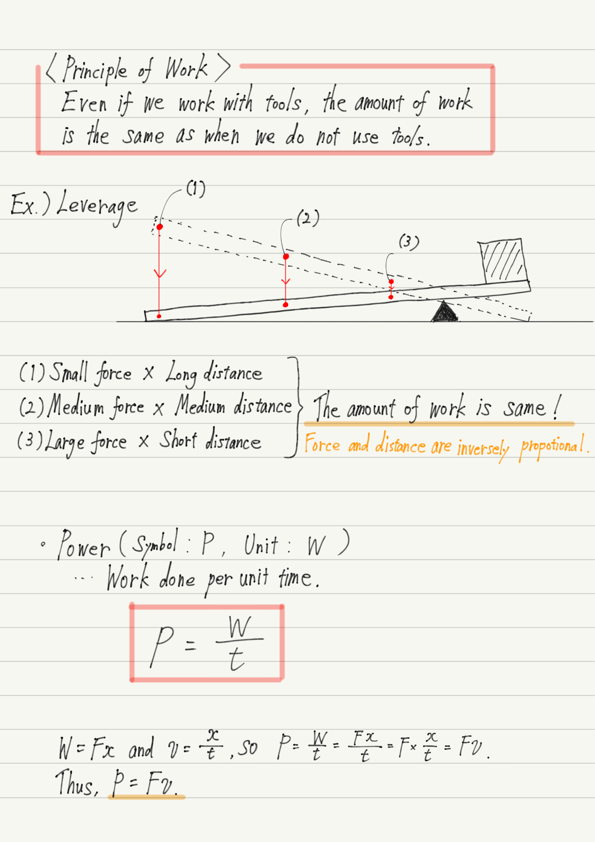# Principle of Work

In this lecture, we will consider work done with tools.
The reason why we use tools when we do some work is, of course, “because it is more convenient to use them.
Let’s consider how they are useful in a physical sense.

## What part of the leverage do you push?

Tools are not complex mechanical devices, but rather primitive tools that are easy to understand.
Textbooks often contain examples of movable pulleys, but here I would like to use a leverage as an example.

Now, consider lifting an object using leverage.
Where would you press for leverage?  Please choose from (1) to (3) below!Probably, many of you chose (1). Why did you choose (1)?

Of course, “because the farther away from the fulcrum, the smaller force is needed”!
That is the “Principle of leverage”.

The principle of leverage is obviously correct, but what I want you to consider again here is, “Is choosing (1) really the best choice?”

Compare the state in which the leverage is pushed to the bottom with the initial state.This figure makes it clear.
Many people choose (1) as soon as they see leverage, but although (1) requires less force to be applied, it requires pushing over a longer distance.
On the other hand, (3) requires a large force but a short distance. (2) is in between the two in terms of both force and distance.

If you choose (1), you can certainly use less force, but considering the disadvantage of the longer pressing distance, I feel that pressing (1) is not the best choice.

## Is work the same everywhere?

We now know that leverage requires us to consider not only the pushing force but also the pushing distance.
Now that force and distance are in play, this is where the work we learned last time (= force x distance) comes in!
The easiest thing for the pusher to do is to push where the work is smallest.

So let’s calculate the work from (1) to (3).

Work at (1) = small force × long distance
Work at (2) = medium force × medium distance
Work at (3) = large force × short distance

This doesn’t quite tell us which is the smallest, but the conclusion is that (1), (2), and (3) are actually all the same value!

When working with tools, if less force is applied, the distance to be moved increases. As a result, the work is the same as when no tools are used.
This is called the “Principle of work”.

This is true for any tool other than leverage, but what is the point of using a tool if it doesn’t change the work?

## Efficiency of work

Suddenly, let us compare Mr. A, who does 600 J of work in 30 seconds, with Mr. B, who does 200 J of work in 20 seconds, to see which is more efficient.
We think in terms of time.

Calculating the work done in 1 second for each, 600 J ÷ 30 s = 20 J for Mr. A.
For Mr. B, 200 J ÷ 20 s = 10 J.

Now you can see at a glance which is more efficient!
The work per second as just calculated is called the “Power”.
The unit is W (watt).
In other words, the power of Mr. A and Mr. B are 20W and 10W, respectively, and it can be judged that Mr. A, whose work rate is larger, is more efficient.

## Leverage problem, again

Now that you have learned the concept of power, let’s return to the first problem.
Where do we push the leverage?

By the principle of work, we have seen that the amount of work remains the same no matter where we push.
But even if the tools don’t change the amount of work you do, they do change the efficiency of your work!

No matter how small the force applied, if the distance to be moved is large, it will take longer to complete the work. The distance to be moved should be as small as possible.

Therefore, if you push “as close to the fulcrum as you can comfortably push”, the work rate will be the greatest, which is a wise use of leverage!

## Summary of this lecture## Next Time

In the next lecture, we will return to the topic of force.

https://www.yukimura-physics.com/entry/dyn-f25

error: Content is protected !!